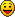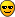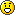Forum » Maths conversation

## Maths conversationVacuus 13 years ago Speaking of the 89, I just bought a Titanium for AU \$120 over ebay..Great, considering my school sells 83+'s for \$200... # quoteAmarth 13 years ago a is right. The others are wrong, I think.For b, keep in mind that you only need to determine the corners. The others don't matter. So don't look at the whole floor, only the corners. Same logic applies as in a.For c, it's a bit different. Of course, you should start with one row, extension to four rows is trivial. What is the chance that a row of four tiles contains at least one white, black and blue tile? # quoteE_net4 13 years ago So? That's the point.Why do you think b) is wrong? # quoteAmarth 13 years ago For b), the chance has nothing to do with having at least 4 blue tiles. The chance is having 4 tiles in 4 positions. If I have 15 blue tiles, but one is white in a corner, then it's a no-go.Also, 1/X^Y/ Z is, apart from ambiguous, just wrong. Assuming standard notation, this is 1/(Z * X^Y), which increases your number of possibilities but not your number of correct ones. Assuming another interpretation, it'd be Z/(X^Y), which is in the right direction of thinking, but Z = 3^4 is wrong then. Z would be, in the set of all possibilities (the 'universe'), the number of those possibilities that are right, and that number is higher.[EDIT] as for C, I don't understand your logic at all. Take in consideration the extra tile that can be any color, and the ordering of the tiles. # quotebwansy 13 years ago "Amarth" said:f(x) = e^x and f(x) = c are walking in the forest. Suddenly, the constant function spots a differential operator. He shouts 'Oh no! If he sees me, I'll be reduced to nothing!" and he runs away. The exponential function goes towards the differential operator and says "I am not scared! You cannot change me, for I am f(x) = e^x!" The differential operator responds: "Hi! I'm df/dy!"Nice one!Kind of reminds me of something I heard someone said (unrelated to maths):"Why are there so many stories about two people walking in the forest and met something horrifying? I mean, in the forest there's only trees, birds, bugs, animals, cavemen drawings, magnetic anomalies and space-time distortions... but no monsters!Here's another joke from a funny TV show which pretended to be serious science education programme:MATHS stands forMathematicalAntiTelharsicHarfatumSeptomin# quoteE_net4 13 years ago Let me revive this thread.My head got a problem, which I can't solve!The relations between the side and the area of a regular polygon with a certain number of sides.I decided to make functions of each regular polygon. Here's what I got:`triangle - A(s) = (s^2 * Sqrt(0.75))/2square - A(s) = s^2pentagon - ...`It was then I stopped. I had to use this formula to get the Area:` A = P/2 * ap<=> A = 5s/2 * ap`Pic:But how if I can't find ap?Argh! Stupid problem. # quoteAmarth 13 years ago You need the sine rule for that. On your drawing, call the center C, the point right beneath it where the ap-line intersects with the side B and the point of the pentagon to the right of it A. In triangle ABC, the sine rule gives`|AB|/(sin C) = |BC|/(sin A)`In this case (try to see for yourself how I got the angles):`(s/2)/(sin 2pi/10) = ap/(sin 9pi/10)`This is generalizable to other regular polygons, the only difference is that your angles will be different. I don't see immediately how you can solve this without using the sine rule, though it might be possible. # quoteE_net4 13 years ago Well, I never learnt that rule, but still... how am I supposed to get the coords of A? # quoteAnonymous1157 13 years ago ... I'd guess that you take the first equation and solve it for A. # quoteAmarth 13 years ago This is not analytical geometry, we're not thinking with coordinates here. Just lengths and angles. I hope you are not confused with my abuse of notation to use the name A for both the point and the angle in the triangle ABC. If I'd have a bit more time, I'd make some drawings. Perhaps tomorrow evening.If you want to use coordinates, I suggest putting A on (0,0) and finding out where everything else is, though I think it's harder. B would be on (0,s/2), you could calculate the direction of BC (you know the angle it makes), so you can create the equation of BC. Then find the point of intersection for AC and BC, and you have the coordinates for C, and thus you know ap, because C is (ap,0).Okay, so that's a way to do it without sine rule. # quoteE_net4 13 years ago Well nice. I must try that. # quoteE_net4 13 years ago Yes, I will try that... Later.As I was thinking about this problem, I had the idea for the following CASIO program:`ViewWindow -1,2,1,-1,1,1Deg"Number of sides"?->AIntg A->AIf a<=2Then 3->AIfEnd360/A->BFor 0->L To A-1cos (LB+(B/2-90))->Csin (LB+(B/2-90))->Dcos ((L-1)B+(B/2-90))->Esin ((L-1)B+(B/2-90))->FF-Line E,F,C,DNext`It isn't so hard to understand it.-> - Stores a value into a list, mat or alpha memory variable. (There's a proper key for this)ViewWindow(Xmin,Xmax,Xscal,Ymin,Ymax,Yscal) - Changes the limits of the window.F-Line X1,Y1,X2,Y2 - Draws a line from (X1,Y1) to (X2,Y2)This program draws a regular polygon with the number of sides you input.If you want to try it and you have no casio calculator, try searching for an emulator. # quoteMageKing17 13 years ago "E_net4" said:`ViewWindow -1,2,1,-1,1,1Deg"Number of sides"?->AIntg A->AIf a<=2Then 3->AIfEnd360/A->BFor 0->L To A-1cos (LB+(B/2-90))->Csin (LB+(B/2-90))->Dcos ((L-1)B+(B/2-90))->Esin ((L-1)B+(B/2-90))->FF-Line E,F,C,DNext`As an interesting side note, this code is easily converted to TI-BASIC by simply removing the "F-" in "F-Line" and storing the window dimensions in Xmin, Xmax, Ymin, Ymax, etc. directly (and changing "IfEnd" to just "End" or "EndIf", depending, and changing "intg" to just "int", and changing around a couple minor syntax quibbles here and there). Oh, and using "Input" or "Prompt" instead of "?->". # quoteE_net4 13 years ago You're totally right.I made another program, by just editing this one:`ViewWindow -1,2,1,-1,1,1 Deg "Number of sides"?->A Intg A->A 360/A->BIf A<1Then 1->AIfEndCircle 0,0,1For 0->L To A-1 cos (LB+(B/2-90))->C sin (LB+(B/2-90))->D 0->E 0->F F-Line E,F,C,D Next`This program divides a circle into X equal parts. # quoteE_net4 13 years ago At last, I've successfully obtained the ways of the Areas of Regular Polygons.And from now on, I shall be called The King of the areas of regular polygons! # quoteAnarion 13 years ago Hey King of the areas of regular polygons, you f**ked your signature up."E_net4's sig" said:[b]I'm the King of the areas of regular polygons![b] # quoteE_net4 13 years ago Time to revive the thread.At the Portuguese Mathematics Olympics of this year, there were 4 Maths problems. Here's the 4th:It is written in ascendant order each multiples of 3, which by adding 1, would make a perfect square number.3, 15, 24, 48, ...Which is the 2006th number written?Let's think about the resolution of it.Sorry if I made any mistakes when translating. # quoteAmarth 13 years ago 1006008? # quoteMageKing17 13 years ago I think you're a little low, unless you're missing a digit somewhere. # quoteAmarth 13 years ago Okay, you are right. How about 9060099? # quoteE_net4 13 years ago You could actually say how you have reached such values! # quoteAnonymous1157 13 years ago "E_net4" said:You could actually say how you have reached such values!I think that'd take up WAY too much space. He could still brush over it, though. # quoteAmarth 13 years ago Easy. We are searching for numbers X of the form N^2 - 1, and where X can be divided by 3. Or, mathematically, N^2 - 1 mod 3 = 0 (technically, not equals, but 'is equivalent with under mod 3' - whatever). We have the following equivalence:`N^2 - 1 mod 3 = 0<=>(N mod 3)^2 - 1 = 0<=>N mod 3 = 1 OR N mod 3 = 2`This means that the Ns make the following sequence: 1, 2, 4, 5, 7, 8, 10, 11, ... Skipping every number divisible by 3.If we look at the problem, we start with the number 3 = 2^2 - 1, so the sequence above actually starts with 2 instead of 1. We can then pick the 2006th number from this sequence of Ns, this is 3010 (check this yourself). The corresponding X is 3010^2 - 1, which one can easily calculate by hand to be 9060099. # quoteMageKing17 13 years ago "Amarth" said:We can then pick the 2006th number from this sequence of Ns, this is 3010 (check this yourself).I actually get 3008."Amarth" said:The corresponding X is 3010^2 - 1, which one can easily calculate by hand to be 9060099.Using my own number (300, the equation is, of course, 3008^2 - 1, calculated to be 9048063.EDIT: I am a moron. As Amarth pointed out, the sequence starts with 2, not 1, so the 2006th number is, indeed, 3010. # quoteAmarth 13 years ago Well, don't bother it. I'm glad my solution is peer-reviewed. There is always the possibility of stupid mistakes (though I don't have to explain that, I think) # quoteE_net4 13 years ago "Amarth" said:We can then pick the 2006th number from this sequence of Ns, this is 3010 (check this yourself).Huh, unfortunately, I didn't manage to complete this step.ops: # quoteAmarth 13 years ago The sequence N(n), being 2, 4, 5, 7, 8, 10, 11, 13, ... can be written as` /N(n) = | 3/2 * n + 1 for n even | 3/2 * (n-1) + 2 for n odd \`N(2006) = 3/2 * 2006 + 1 = 3010. # quoteE_net4 13 years ago Oh.. I see.Here's the 3rd problem:The numbers 1, 2, 3,... are sequentially written on a (100 characters x 100 characters) screen, with a space between the numbers. When there's no room for the next number, that number is written at the beginning of the next line.Which is the last written number on the screen? # quoteAmarth 13 years ago 2140? Not at all certain, might be off by a couple. My reasoning is pretty straightforward, let's see what others come up with...[EDIT]Wait... Is the space dropped if the number is at the end of the line? Not sure it matters, but it's kind of ambiguous. # quoteE_net4 13 years ago Well, it says: "When, in the end of the line, there's no space to write another number, it is wirtten at the beginning of the next line."And so this rule does only apply to the number. # quoteMageKing17 13 years ago "Amarth" said:The sequence N(n), being 2, 4, 5, 7, 8, 10, 11, 13, ... can be written as` /N(n) = | 3/2 * n + 1 for n even | 3/2 * (n-1) + 2 for n odd \`N(2006) = 3/2 * 2006 + 1 = 3010.Or my simpler solution,`N(n) = ceiling( 3 / 2 * n ) - 1`but really, either way works.N(2006) = ceiling( 3 / 2 * 2006 ) = 3010 # quoteE_net4 12 years ago Recently, I've been learning Trigonometry in Maths class and I came upon a dilema...Is it admissible that tan(pi/2) = Infinite ? Well, there are reasons to say this, but is this expression valid? # quoteAmarth 12 years ago Sigh. # quoteIdiota 12 years ago Indeed, that's some basic stuff you're pulling man... # quoteE_net4 12 years ago I came on an urge to come to this thread, for I bring an invention and a problem of mine.Let's start on the problem.I have a trinangle, whose 3 vertices' coords are known. What I need to get from these is the equation that defines all points inside the triangle.Here's something for you to understand the problem. I need to know the equation that tells me if a point belongs to the grey area of the picture:This would be useful on computer graphics, where I could draw 2D triangles.Now for the most interesting part.Once upon a night, I was holding some paper, doing some random mathematical stuff, before I went to sleep. At that moment I wrote an empty byte:00000000And I implemented a rule: the bit whose surrounding bits were both equal to it, would be toggled. So, the 0 with two 0 "neighbours" would become 1:01111110I proceeded with this rule, but changed one factor: the bit would now have to have exactly one equal neighbour. The result:00111100And then I toggled the neighbour count again to 2, just like the beginning of this sequence:00100100Toggled again to 1 neighbour:11111111Toggled(2):10000001Toggled again(1):11000011Toggled again(2):11011011And curiously, the next value of the sequence is:00000000Which forms an infinite sequence.The total of the sequence, including the convertions:` BIN -> DEC -> HEX00000000 -> 0 -> 001111110 -> 126 -> 7E00111100 -> 60 -> 3C00100100 -> 36 -> 2411111111 -> 255 -> FF10000001 -> 129 -> 8111000011 -> 195 -> C311011011 -> 219 -> DB`Bitmap of the sequence (8x scale):Now I'd just like you to tell me what you think about it. I don't even know whether anyone found out this sequence... # quote
Forum » Maths conversationYour email: Your name: